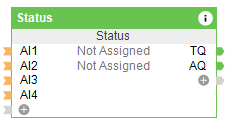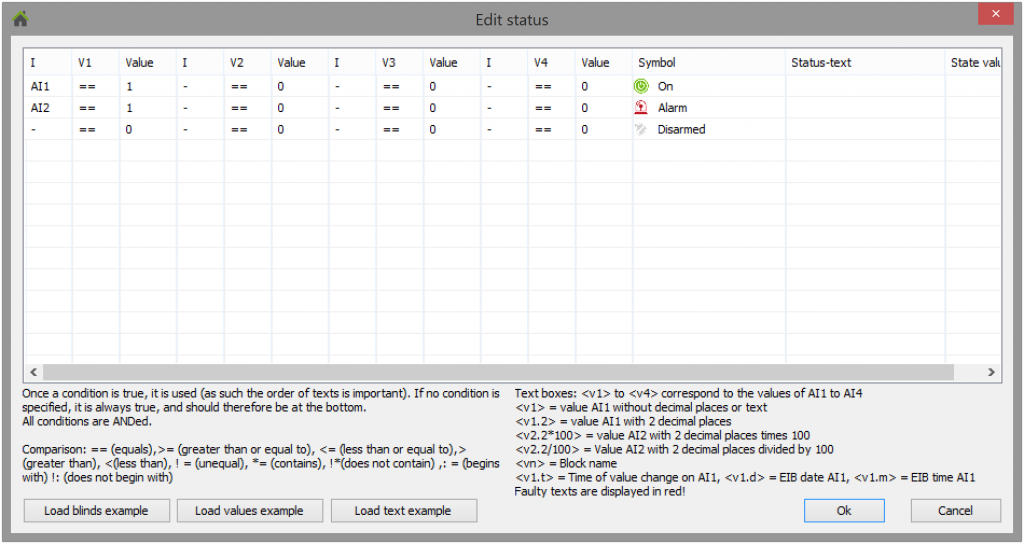# Status

## APPLICATION

With the Status function block, you can visualize custom status texts and symbols.

Furthermore, this can be used for decision logics.

## BASIC SETUPBy double-clicking on the program block the processing window can be opened.In this dialog, conditions can be defined and the output which is displayed when the conditions are met.

The order of the entries is important. Once all the conditions in a row are met, the remaining rows are no longer checked and the output of this line is output.

### Conditions

A maximum of 4 conditions per line can be inserted.

A condition consists of: selected input, comparison operator, value

When ALL conditions are entered in one line, the following status text, status value and the symbol are output in the visualization and in the outputs.

### Possible conditions

 == Is equal to the value > Is greater than the value >= Is greater than or equal to the value < Is less than the value <= Is less than or equal to the value != Is not equal to the value *= Selected input contains the value !* Selected input does not contain the value : The selected input begins with the value !: Selected input does not start with the value

### Possible issues

In general, there are 3 output options:

1. Symbol: Here the predefined symbols can be selected. The icon is then displayed in the visualization.
2. Status text: Here, text output is possible. The text is displayed later at the output TQ and also in the visualization.
3. Status value: Here analog value outputs are possible. The value is displayed at output AQ.

With the status text the value of the input can be processed further. For this purpose, a placeholder must be inserted at the position at which the value of the input is to be set. The following is a list of all possibilities to process the applied value further:

 Placeholder Operation Example Result example (AI1 = 5,382) X = Input number (Ex: ) The value at the input is inserted at this point in the text The outside temperature is degrees. The outside temperature is 5.382 degrees X = Input number (Ex: ) The value at the input is inserted at this point in the text with Y decimal places The outside temperature is degrees. The outside temperature is 5.38 degrees X = input number (Ex: ) The value of the input is multiplied by Z and inserted at this point in the text with Y decimal places The outside temperature is degrees. The outside temperature is 16.15 degrees X = input number (Ex: ) The value of the input is divided by Z and inserted at this point in the text with Y decimal places The outside temperature is degrees. The outside temperature is 1.79 degrees Name of the status block Our status module is called “” Our status module is called “outside temperature” Time of change from input X The last temperature change was The last temperature change was 2016-12-22 16:31:24 Converts the analog value at input X to an EIB datum. The current date is The current date is 2005-00-00 Converts the analog value at input X to EIB time. The current date is The current time is 00:00:05